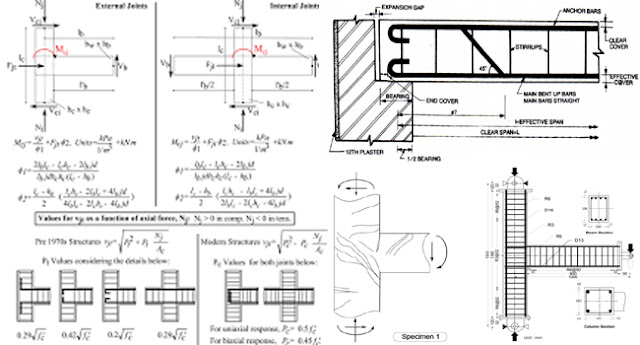Very useful construction tips to design a rectangular beam

# Very useful construction tips to design a rectangular beamThis construction video sifts through the phases which are essential to create the design of a rectangular beam.
The total steps are divided as follow :-
The first step – Process for detecting the depth of beam :-
The Formula for Depth of beam is = l/16 (Here l stands for the length or span and 16 stands for constitute Lu.
The second step – Process for detecting the breath of beam :-
The formula for Breath of beam = 50 percent to 60 percent of depth of beam
The third step – Process for figuring out self weight of the beam :-
The formula for self weight of beam = S.W = B x d x density of concrete
Here B denotes breath, d denotes depth and S.W denotes self weight
Therefore, Weight (W) = 145 to 154 lbs/ft3 or Cft.

Continue Reading about the "Very useful construction tips to design a rectangular beam" on the next page below

1.1.